﻿ FSS单元排布对天线罩电磁波散射的影响 The Influence of FSS Unit Arrangement on Electromagnetic Scattering of Radome

Applied Physics
Vol. 09  No. 12 ( 2019 ), Article ID: 33264 , 8 pages
10.12677/APP.2019.912060

The Influence of FSS Unit Arrangement on Electromagnetic Scattering of Radome

Xiangyu Li, Xiaolong Huang, Miao Yu, Jianbo Wang*

College of Science, Changchun University of Science and Technology, Changchun Jilin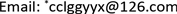Received: Nov. 11th, 2019; accepted: Nov. 27th, 2019; published: Dec. 4th, 2019ABSTRACT

In the actual application and design process of frequency selective surface (FSS), it is inevitable to encounter the problem of arranging unit structure on the curved surface, which is different from the infinite plane model in simulation. In this paper, based on the actual engineering and focusing on the problems in the real situation, we study the influence of unit structure arrangement on the electromagnetic scattering characteristics of a straight conical radome based on the circular ring structure by using EastFDTD software. Through comparison and analysis of the three layout methods, it is found that the optimal layout after eliminating the defects is beneficial to the electromagnetic scattering characteristics of the radome, which can better realize the free permeability of the electromagnetic wave in the working frequency band and the high reflection in the non-working frequency band.

Keywords:FSS, Radar Cross Section, Unit Structure Arrangement, Electromagnetic Scattering

FSS单元排布对天线罩电磁波散射的影响1. 引言

2. 单元设计与仿真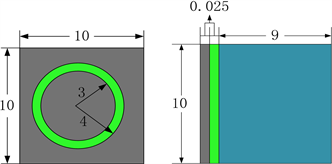(a) (b)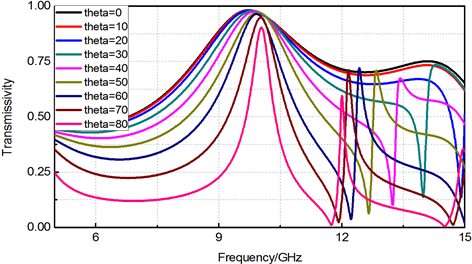(c)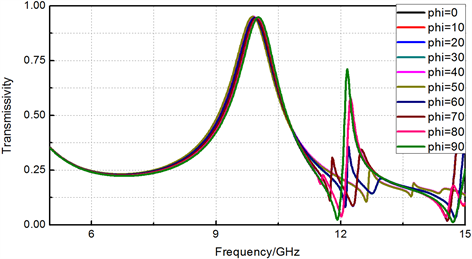(d)

Figure 1. (a) (b) Elevation and side views of the unit structure, unit(mm); (c) Transmittance curve of the unit model when the polarization angle is fixed and the incidence angle is changed; (d) Transmittance curve of the unit model when the incidence angle of the fixed polarization angle changes

3. 天线罩的仿真与分析

3.1. 无FSS加成的天线罩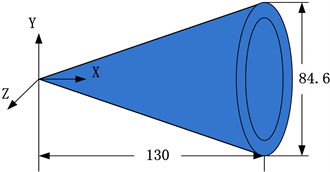Figure 2. A three-dimensional schematic of the radome (unit: mm)

3.2. 平面FSS排布与仿真(a)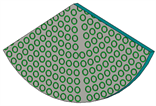(b)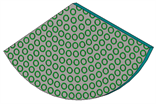(c)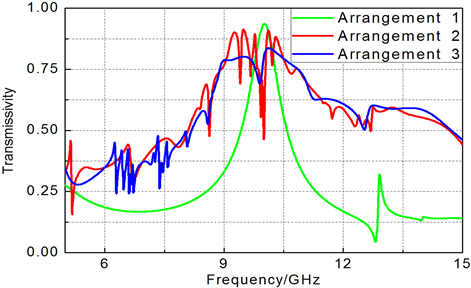(d)

Figure 3. (a)~(c) The straight cone model is developed into a schematic diagram of plane fan, which is arranged one, two and three respectively in order; (d) Transmittance curves of three models in 5 - 15 GHz

3.3. 直锥模型的FSS排布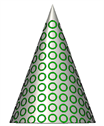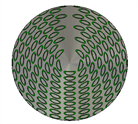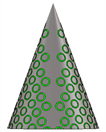(a)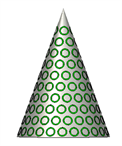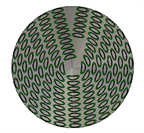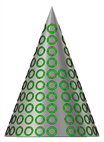(b)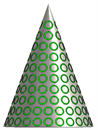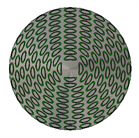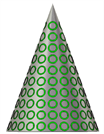(c)

Figure 4. Front view, top view and rear view of radome, (a) is the model view of arrangement one; (b) is the model view of arrangement two; (c) is the model view of arrangement three

3.4. 直锥模型的FSS仿真数据

3.4.1. 缺陷部分的讨论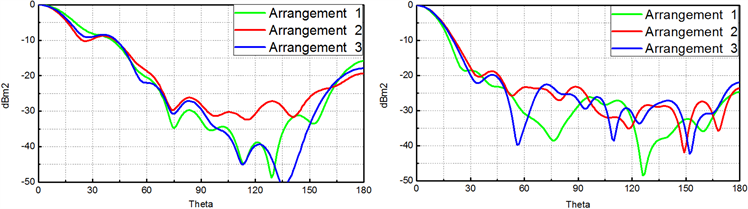(a) (b)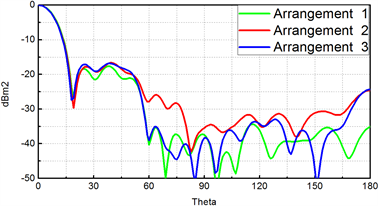(c)

Figure 5. Electromagnetic wave scattering curves of three kinds of models at different frequencies in defect section, (a) is the electromagnetic wave scattering curve at 7 GHz; (b) is the electromagnetic wave scattering curve at 10 GHz; (c) is the electromagnetic wave scattering curve at 13 GHz

3.4.2. 非缺陷部分的讨论

3.5. 小结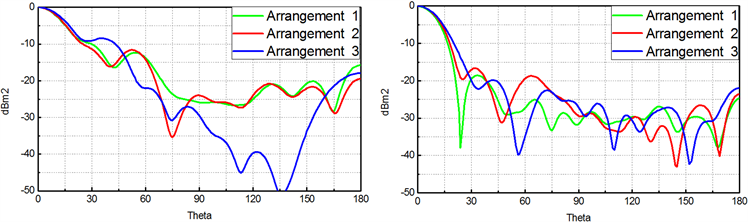(a) (b)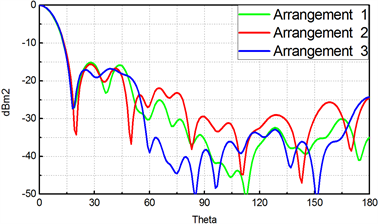(c)

Figure 6. Electromagnetic wave scattering curves of three kinds of models at different frequencies in the non-defective section, (a) is the electromagnetic wave scattering curve at 7 GHz; (b) is the electromagnetic wave scattering curve at 10 GHz; (c) is the electromagnetic wave scattering curve at 13 GHz

4. 总结

The Influence of FSS Unit Arrangement on Electromagnetic Scattering of Radome[J]. 应用物理, 2019, 09(12): 491-498. https://doi.org/10.12677/APP.2019.912060

1. 1. 桑建华, 编著. 飞行器隐身技术[M]. 北京: 航空工业出版社, 2013.

2. 2. D. Richardson著. 现代隐身飞机[M]. 魏志祥, 等, 译. 北京: 科学出版社, 1991.

3. 3. 阮颖铮, 等, 著. 雷达截面与隐身技术[M]. 北京: 国防工业出版社, 1998.

4. 4. 王佳欢, 汪剑波. 特定曲面频率选择表面的电磁散射特性研究[D]: [硕士学位论文]. 长春: 长春理工大学, 2014.

5. 5. 司薇, 卢俊. 有源频率选择表面传输特性的研究[D]: [硕士学位论文]. 长春: 长春理工大学, 2014.

6. 6. 周龙建. 平台天线一体化宽带隐身关键技术研究[D]: [硕士学位论文]. 成都: 电子科技大学, 2017.

7. 7. Sipns, Z., Bosiljevac, M. and Bartolic, J. (2008) Rigorous and Approximate Analysis of Curved Frequency Selective Surfaces. IEEE: Antennas and Propagation Society International Symposium, San Diego, CA, 5-11 July 2008, 1-4. https://doi.org/10.1109/APS.2008.4619216

8. 8. 吴楠, 等. 排列方式对Y形单元厚屏频率选择表面传输特性的影响[J]. 长春理工大学学报, 2013, 36(6): 116-119.

9. 9. Munk, B.A. (2009) Frequency-Selective Surfaces: Theory and Design. Wiley, New York.

10. 10. 朱军, 等. 曲面频选天线罩电性能设计研究[J]. 微波学报, 2019, 35(1): 69-74.

11. 11. 王向峰, 高炳攀, 等. 一体化曲面共形频率选择表面雷达罩[J]. 光学精密工程, 2018, 26(6): 1362-1369.

12. 12. 陈毅乔. 曲面双层带通频率选择表面天线罩设计[J]. 电讯技术, 2016, 56(4): 458-462.